Contact

• Andrius Kulikauskas
• ms@ms.lt
• +370 607 27 665
• Eičiūnų km, Alytaus raj, Lithuania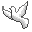My original work is in the Public Domain for you to use in your best judgement.

Support me!

• Patreon...
• Paypal to ms@ms.lt
•BookshelfAs an Amazon Associate I earn from qualifying purchases.

Thank you!

Latest Math

2021.05.02 I am finding different angles for intuitively understanding the classification of orthogonal polynomials:

• Weights?
• Moments
• Hankel determinants
• Combinatorial objects
• Zeng nurseries of trees with links and kinks
• Probability distributions

I think these variously manifest a fivefold classification that is the cognitive framework which is inherent in decision making and thus human conceptions of time and space. I am exploring the insights this can yield into solutions of the Schroedinger equation, and thus the workings of quantum physics, the paradigms available for it, and also their interrelationships.

Given a family of orthogonal polynomials {$\{P_n(x)\}$}, a key fact is that any polynomial {$f(x)$} can be uniquely expressed as a linear combination of them. And so, in particular, we can consider {$xP_n(x)$}, and realize that its expression may not include any {$P_k(x)$} where {$k>n+1$} or where {$k<n-1$}. If the orthogonal polynomials are monic, such that the leading term is {$x^n$} with coefficient {$1$}, this means we have {$xP_n(x)=P_{n+1}(x)+A_nP_n(x)+B_nP_{n-1}(x)$}. Here we see that the variable {$x$} comes from a single source on the left hand side but that it impacts the polynomial just above and the one just below in the hierarchy. The upshot is that the exponential possibilities are tamed by a second order recurrence relation, or alternatively, a second order differential equation. These quadratic fetters yield a fivefold classification based on the discriminant {$\sqrt{b^2-4ac}$}, much as with conics.

For example...

• {$\int P_n(x)P_k(x) dw = c_k\delta_{nk}$}
• {$f(x)=f_0P_0(x)+f_1P_1(x)+\cdots+f_{n-1}P_{n-1}(x)$}
• {$\int P_n(x)f(x) dw = 0$}
• look at {$\int xP_n(x)P_k(x) dw =$} this becomes an argument about P_n, and it says suppose that n>=k, then because xP_k(x) has degree k+1, so if n>k+1, which means n-1 > k, then it will be zero.

I am studying how to derive Sheffer's fivefold classification of orthogonal polynomials of A-type 0. This fivefold classification variously interprets the generator for walks with two kinds of step, {$\alpha$} and {$\beta$},

{$$\frac{1}{(1-\alpha t)(1-\beta t)}=(1+\alpha t+\alpha^2t^2+\cdots)(1+\beta t+\beta^2t^2+\cdots)$$}

where we may have

• no steps {$\beta = \alpha = 0$} (Hermite)
• one kind of step
• {$\beta = 0$}, {$\alpha \neq 0$} (Charlier)
• {$\beta = \alpha \neq 0$} (Laguerre)
• two kinds of step
• both real: {$\beta < \alpha$} (Meixner)
• complex conjugates: {$\beta = \overline{\alpha}$} (Meixner-Pollaczek), so that {$\alpha=a+bi$} and {$\beta=a-bi$}

But where do {$\alpha$} and {$\beta$} come from? What do they mean mathematically, physically, cognitively? I have found helpful materials including atreasure trove of lectures by Xavier Viennot on the combinatorics.

2021.04.25 I suspect that strings of adjoint functors may express what I mean by divisions of everything. I am thus trying to thoroughly understand the concept of adjunction in category theory, which is also important for truly mastering the Yoneda Lemma. I am therefore studying how to relate the three definitions of adjunction in terms of homsets, units and counits, and the universal mapping property. I have gone through the six ways of going from one definition to another. They all seem to serve to invert a functor in a given context. I think they also function like pushdown automata, for example, satisfying obligations, such as matching left parentheses with right parentheses. Now I am trying to work out and understand what that means for particular adjunctions such as inclusion for preorders.

See also my diary and the recently changed pages in my Exposition and Research sections.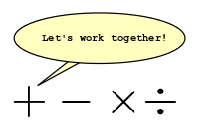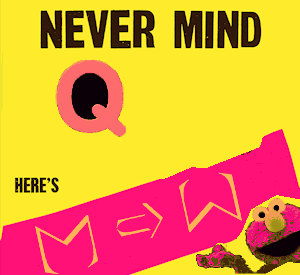Truthful Wisdom

{$M\Rightarrow W$}, "M 4 W" and Math 4 Wisdom stand for Mathematics for Wisdom by Andrius Kulikauskas, ms@ms.lt, aka Living by Truth.

{$M\Rightarrow W$} inspires our imagination by studying its very limits, what we can imagine or not. Let's embrace the cognitive frameworks we can't escape and leverage them for a conceptual language of wisdom that we can express, validate and apply with the help of mathematics and all manner of sciences, creative arts and moral disciplines.

Cranky Disclaimer

You may be at risk of dismissing these pages as an online art show, science fiction, mental poetry, psychological symptoms, products of numerology, metaphysical truths, existential crises, adventures in mysticism, primordial forms... and so on and so on and so on. Just so you know!

Keys to Math and Other Subjects

Coming sooner or later...

Profoundly Speculative Investigations

Why study math? Here are mathematical concepts that I believe are relevant for wisdom.

 Understanding for modeling Mathematics Wisdom The ways of figuring things out in mathematics House of Knowledge for Mathematics Four classical Lie families Four levels of geometry and logic Polytopes Choice frameworks {$SU(2)$} Emotional transformations Yoneda lemma Division of everything into four levels of knowledge (Whether, What, How, Why) Yates Index theorem Division of everything into four... The five conics Division of everything into five perspectives for decision making (thus space and time) Fivefold classification of Sheffer polynomials of A-type zero Division of everything into five... Adjoint string of length N Division of everything into N perspectives Bott periodicity Eight-cycle of divisions Grothendieck's six operations Six representations of divisions Perturbation Intervention, intercession, miracle Spinor Going beyond oneself into oneself {$F_1$} Division of everything into 0 perspectives The state of contradiction God

Wonderfully Curious Phenomena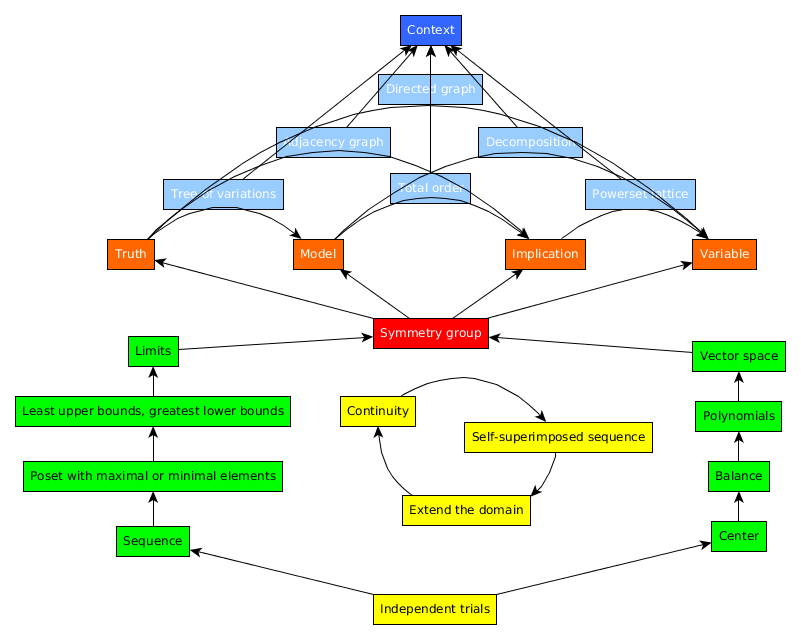24 ways of figuring things out.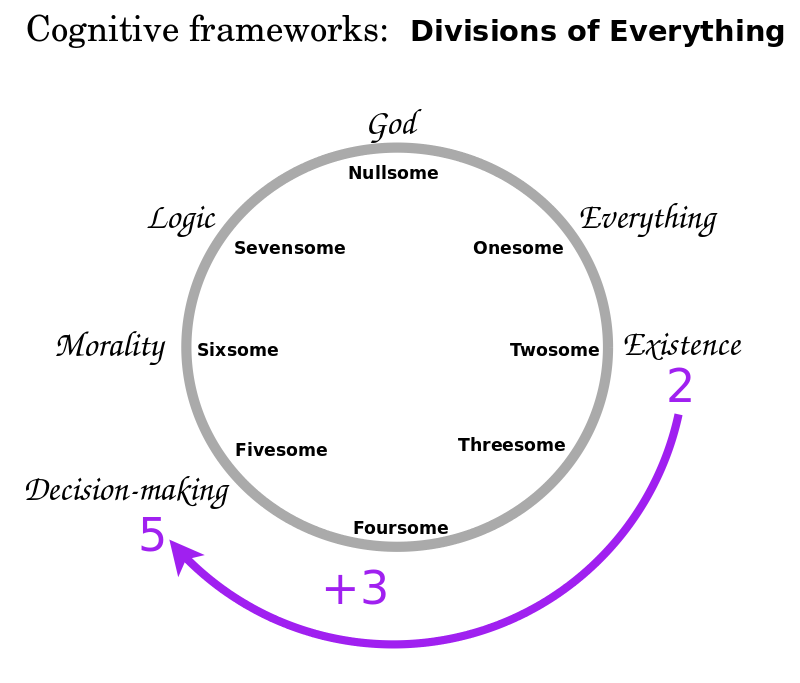8 states of mind.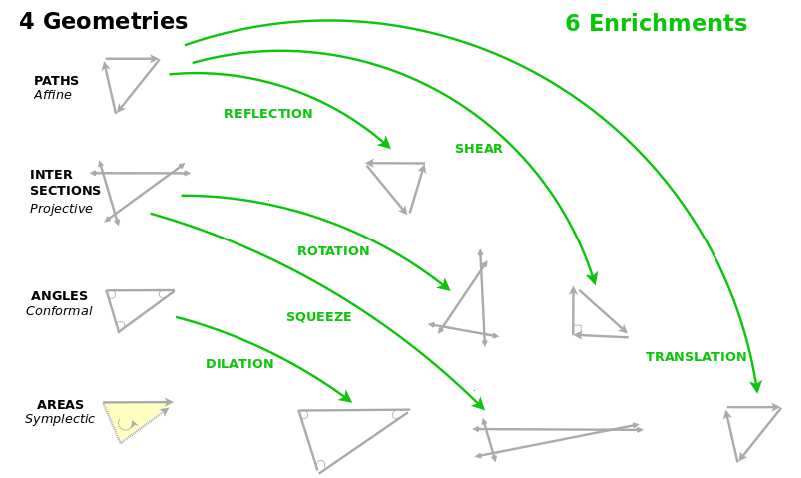6 transformations of mood.

In 1971, as a six-year old child, I went to God (?) with my quest to know everything (?) and apply that knowledge usefully (?)

In 2021, I am about ready to share my wisdom (?) But who would (?) how could (?) why should anybody make an effort to comprehend it (?) take it to heart (?) try it out (?) and work together (?)

Math is a standard for truth. Math is verified again and ever again. It is a universal language, accessible to millions who make the effort, regardless of their personal creed. Enthusiasts, practitioners and specialists appreciate profound results and helpful expositions. They will invest themselves to understand, if they have good reason.

I think it best to demonstrate my wisdom through math:

• to apply my wisdom by overviewing all of math, showing how it unfolds, with all of its branches, concepts, questions and results.
• to express in mathematical form the cognitive frameworks that capture my wisdom.
• to develop insightful expositions of the relevant mathematics.
• to present my work-in-progress as I pursue my ideas in life and math.

Currently, for a year or two, I have the means to spend all of my time on my philosophy and related mathematics. But then I will need to find a way to make a living. My plan is to create this website as a work-in-progress where I present the math that I have learned, am learning or will be learning which is relevant for sharing my wisdom. I think that I will find supporters, including through Patreon, who would fund me to learn and present more math because they love advanced mathematics, want new ways of understanding it, wish to see it reach more people, and are excited how it might serve to express answers to life's great questions. I can also create learning materials, crowd source projects at Kickstarter, and work as a coach for self learners. As ever, my creative work is in the Public Domain, for all to use as they think best.

Ultimately, I hope that my wisdom grows to become our wisdom, the Holy Spirit in a language, a science, a culture of self learners. This website may reach you in a way that academic peer reviewed articles never would. I may write such articles, but I fear they won't be read, understood, embraced and lived. I can write here to you in a way that I would write to myself.

It seems natural to illustrate my ideas with visual thinking, combining text, images and diagrams, as I learned from John Rogers and from Robert Horn's books. Moreover, art is fun, art is social, art holds us together, art changes the rules, so that you might give me a chance. I want to speak from all of me, my conscious and unconscious, to all of you, your conscious and unconscious. I want you to feel that I am a person, real and true, who stands alone, yet aims to commune with you.

Wisdom is why I write, math is how I write. What I write are the creative arts that speak to your attention. Community with you is whether I succeed in writing to you that Spirit which reaches you, and whether from you it reaches me, so that it dances amongst us, as we step-in and step-out, as inspired.

An Introduction to Everything

Coming soon...

...

...

{$M\Rightarrow W$}, Math 4 Wisdom and Math for Wisdom stand for Mathematics for Wisdom by Andrius Kulikauskas.

Šis puslapis paskutinį kartą keistas May 04, 2021, at 04:22 PM